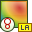# OF Card

This card specifies an offset for the origin of the coordinate system for near and far field calculations. It also facilitates using only a part of the structure through label selection when calculating fields.

On the Request tab, in the Solution requests group, click theRequest options (OF) icon.

## Parameters:

Field calculation for all structures
Near and far fields are calculated for all structures.
Field calculation for structures with specified labels(s)
Use label selection when calculating near and far fields. Only the currents on structures with specified labels are used during field computation.
Field calculation for structures with label range
Use label selection when calculating near and far fields. Only the currents on structures with a label in the range specified in the fields Start at label and End at label are used during field computation. (If a basis function extends over, for example, two triangles it is included if either triangle is in the specified range.)
Calculate near field or far field in local coordinate system
Select this option to calculate fields in a local coordinate system. Define the local coordinate system by specifying the offset from the origin and the rotation about the axis.
Origin of offset coordinate
Specify the Cartesian coordinates of the transformed origin. These values are affected by the scale factor of the SF card if used.
The angle of rotation ${\alpha }_{x}$ around the X 軸, the angle of rotation ${\alpha }_{y}$ around the Y 軸 and the angle of rotation ${\alpha }_{z}$ around the Z 軸 in degrees.